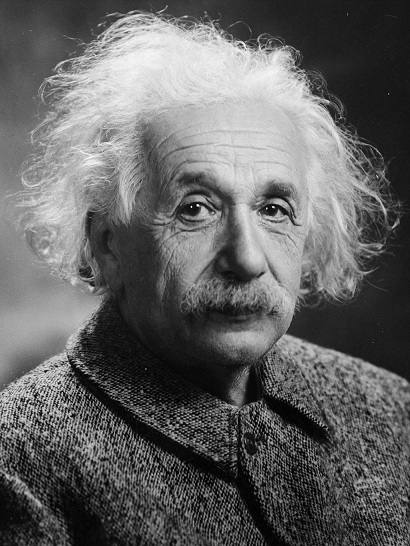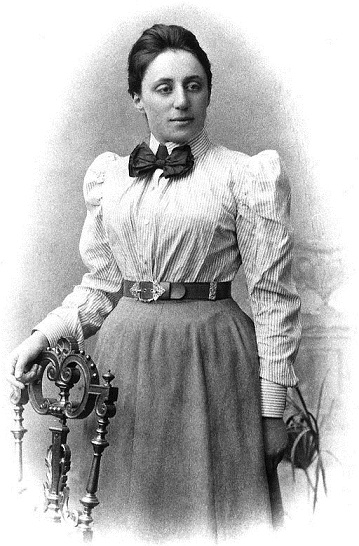# Difference Between Energy and Matter

## Main Difference – Energy vs. Matter

Energy and matter are two fundamental concepts in physics. These concepts are deep and often abstract. Therefore, it is not possible to make a clear, direct comparison. Classically, matter is “defined” as “anything that has mass and occupies space” while energy is described as “the ability to do work”. However, these are not complete descriptions. At a basic level, we could say that the main difference between energy and matter is that matter refers to “objects” whereas energy refers to a property that an object could have .

## What is Matter

It is difficult to give a straightforward definition to “matter”. As mentioned above, matter has been traditionally described as “things that have mass and occupy space”. However, there are several issues with taking this to be a strict definition. For instance, in quantum mechanics there are situations where two particles could technically occupy the same space. On the other hand, there are force-exchange particles that have mass (such as W and Z bosons) which are not often thought of as “matter”. For the purpose of this article, we will consider matter to be things made of quarks and leptons (again, this should not be taken seriously because it potentially excludes dark matter!).

## What is Energy

Broadly speaking, energy can be divided into three types: mass-energy, kinetic energy, and potential energy. Mass-energy refers to the energy associated with the mass of an object. If an object has a mass$m$, then the energy$E$ associated with that mass is given by Einstein’s famous equation describing mass-energy equivalence:$E=mc^2$

where$c$ is the speed of light in a vacuum.Einstein discovered the fundamental relationship between mass and energy.

Kinetic energy is the energy that an object has due to its motion. The faster an object moves, the more kinetic energy it has. In classical physics, kinetic energy is often given by$\frac{1}{2}mv^2$. However, in relativity, kinetic energy is defined in terms of the object’s momentum,$p$.

Potential energy is the energy that a particle has due to the way it interacts with other particles. Different types of potential energy include gravitational potential energy (for massive particles in gravitational fields) and electrical potential energy (for charged particles in electric fields).

The total energy$E$ for a free particle—i.e. a particle that does not interact (so that it has no energy in the form of potential energy), is given by the sum of mass and kinetic energies:$E^2={\left( mc^2\right)}^{2}+{\left( pc\right)}^{2}$

Arguably, the most important concept in classical physics is the law of conservation of energy. This states that the total energy in an isolated system is conserved. This means that energy cannot be created or destroyed, but rather it can be only converted from one form to another. Although the statement sounds qualitative, it has firm mathematical roots in Noether’s theorem developed by the German mathematician Emmy Nother.Work by Emmy Noether (1882 – 1935) helped reveal the mathematical laws underlying the conservation of energy.

## Difference Between Energy and Matter

### Basic Definition:

Matter describes an object

Energy describes a property that an object possesses.

### Classical Definition:

Traditionally, matter has been described as “things that have mass and occupy space”.

Energy has been described as “the ability to do work”.

References:

1. Strassler, M. (2012, April 12). Matter and Energy: A False Dichotomy. Retrieved September 29, 2015, from Of Particular Significance:

Image Courtesy

“Albert Einstein” by Oren Jack Turner (United States Library of Congress) [Public Domain], via Wikimedia Commons

“Portrait of Emmy Noether”, author unknown [Public Domain], via Wikimedia Commons